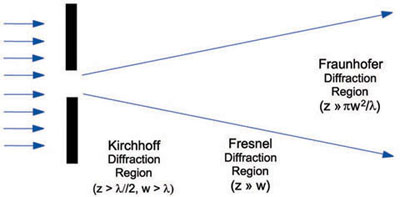# Diffraction

Excerpt from Field Guide to Optical Lithography

Diffraction is usually thought of as the bending of light as it passes by an edge. More correctly, diffraction theory simply describes how light propagates. This propagation includes the effects of the surroundings (boundaries).

A simple interpretation of the physical principle behind diffraction is best captured by Huygen's Principle: any wavefront can be thought of as a collection of radiating point sources. The new wavefront at some later time can be constructed by summing up the wavefronts from all of the radiated spherical waves. Joseph Fresnel formed a mathematical theory of diffraction by turning this summation into an integral and including the phase of the light when adding together the propagating spherical waves. This scalar diffraction theory was put on a more rigorous footing by the mathematician Gustav Kirchhoff, who required the diffracting waves to satisfy the Helmholtz equation and conservation of energy. Fresnel's formulas are in fact a simplification of Kirchhoff's formulation for the case when the distance away from the diffracting plane (that is, the distance from the mask to the objective lens) is much greater than the wavelength of light. Finally, if the distance to the objective lens is very large, or if the mask is illuminated by a spherical wave which converges to a point at the entrance to the objective lens, Fresnel diffraction simplifies to Fraunhofer diffraction.Citation:

C. A. Mack, Field Guide to Optical Lithography, SPIE Press, Bellingham, WA (2006).

View SPIE terms of use.
Excerpt from
Member: \$35.70
Non-Member: \$42.00PREMIUM CONTENT
Sign in to read the full article
Create a free SPIE account to get access to
premium articles and original research
Forgot your username?